# Teaching Methodology# Structured Derivations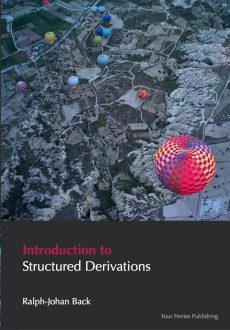### Structured Derivations

Structured derivations is a method for presenting mathematical arguments in a precise and easily understandable form. Structured derivations make it easier for students to follow and understand when the teacher presents a mathematical argument. It gives the students a template for how to construct their own solutions to mathematical problems. The uniform presentation format makes it easy to check and find errors in students' solutions. A structured derivation can also be analyzed by a computer,  to check that the derivation is meaningful and that each step in the derivation is mathematically correct.

The format can be used for all kinds of mathematical arguments: calculations, solving equations, simplifying expression, proving theorems, geometrical constructions, and so on. Structured derivations can be used at any level of mathematics, from pre-algebra to university level and research, and can be used in any area of mathematics.

A simple calculation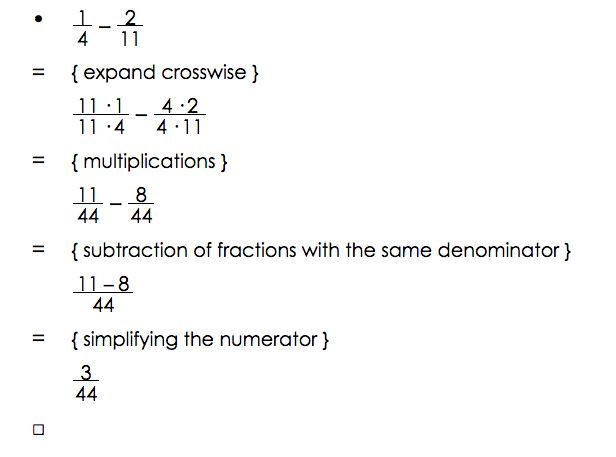### Editor for Structured Derivations

The structured derivations editor makes is easy to construct derivations. It is a syntax-oriented editor, so it guides the user into creating a structured derivation with the correct syntax.  The editor supports standard mathematical notation, both for creating mathematical formulas and for editing these. It can be used in any area of mathematics.

The editor shows you the editing operations that are allowed at each place in the derivation. You can add derivations steps, remove derivation steps, and edit derivation steps at anywhere. Mathematical symbols can be written using LaTeX shortcuts or picked from a symbol palette.

In a calculation step, the editor first creates a copy of the previous term. This makes it easy to make small changes in large formulas, and reduces typing errors in the derivation. The editor can selectively show and hide nested derivations. Hiding the nested derivations allows you to see the overall argument, showing a nested derivation allows you to focus on details. The structured derivations editor has a plug-in for automatically checking the correctness of the derivation.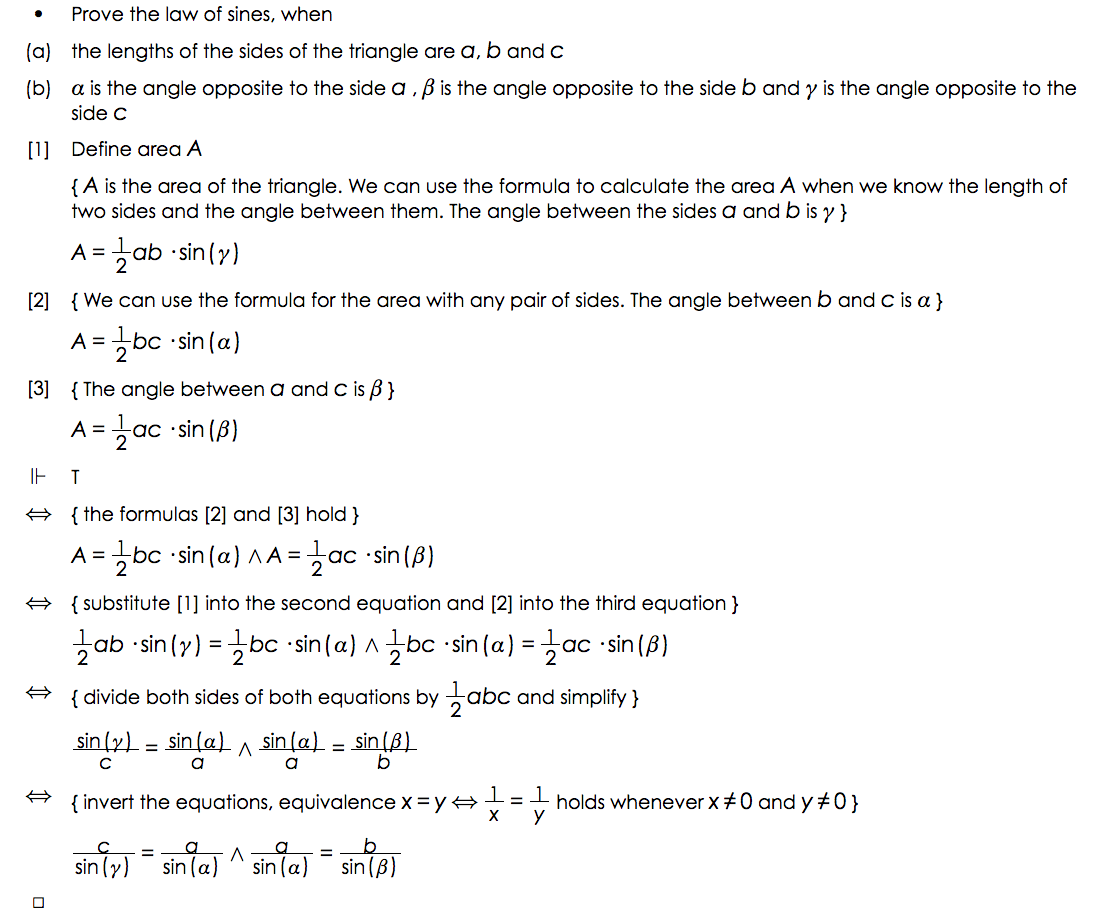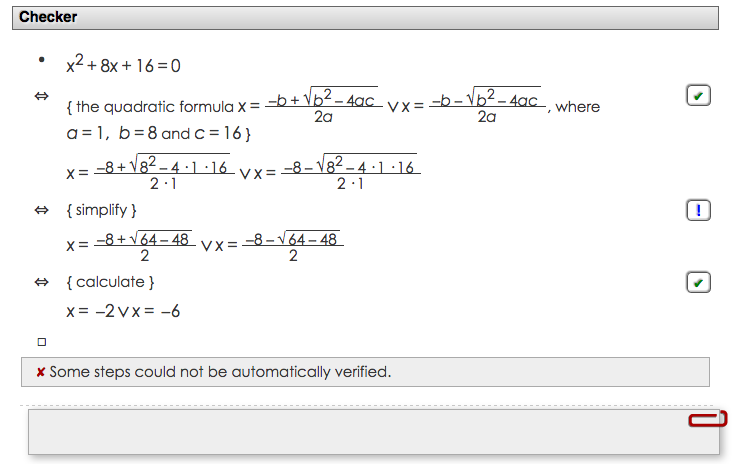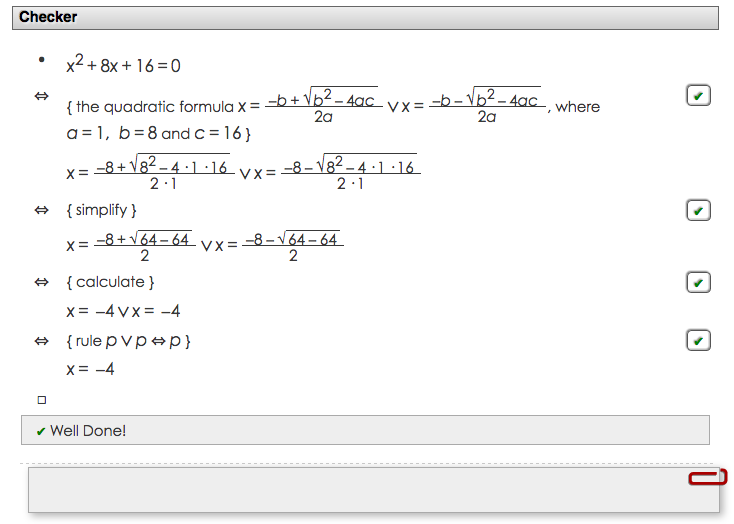### Automatic Checking

A structured derivation can be checked for correctness by a computer. The checker goes through your derivation step by step, marks all steps that are mathematically correct,  and points out the weak spots and potential problems in your mathematical arguments.  The first picture shows a derivation with an error, the second picture shows the checking result after the error has been corrected. Read more ...

### eMath Textbooks

The eMath textbookseMath 1 - eMath 10,  cover all topics in high school mathematics (grades 10 - 12). The books are structured according to the Finnish national curriculum for high school math, but can be used also as references in other national curriculum,  the content of high school math is more or less the same all over the world. The books are available in three languages, Finnish, Swedish, and English. The books all use structured derivations for presenting different kinds of mathematical arguments, like proofs, calculations, geometric constructions, and general problem solving. The books contain a lot of assignments, which the students can solve directly in the book using the eMath editors available in 4f Studio. Read more...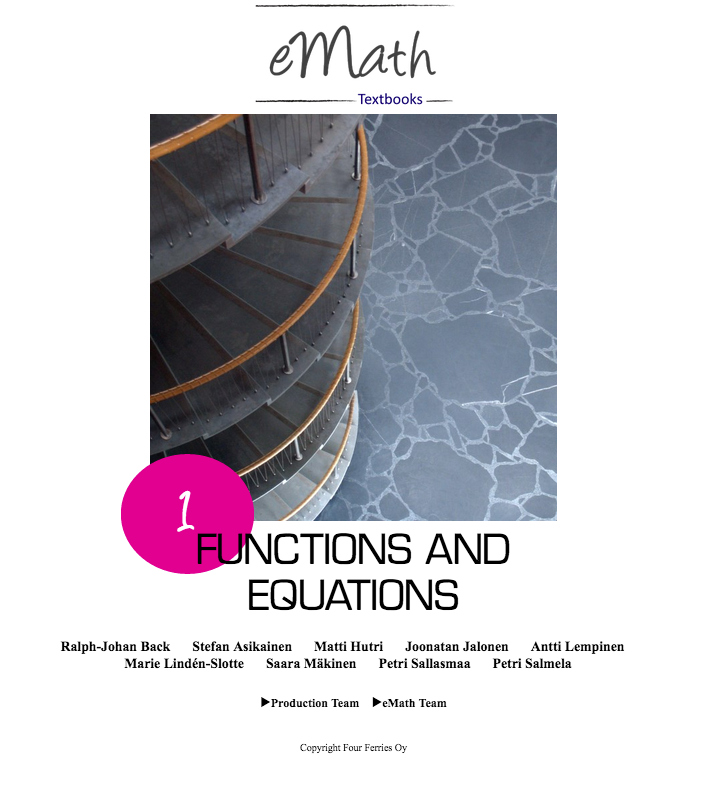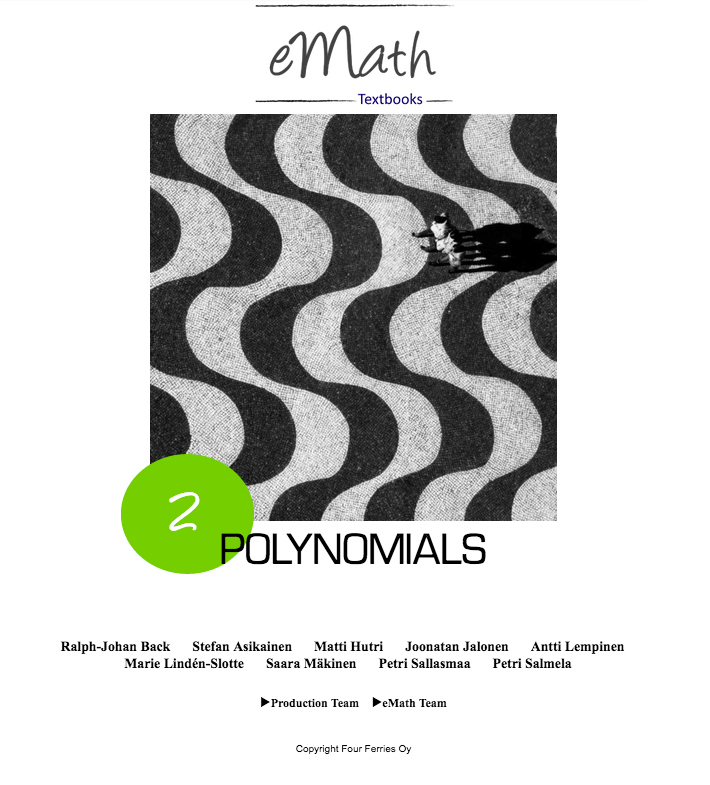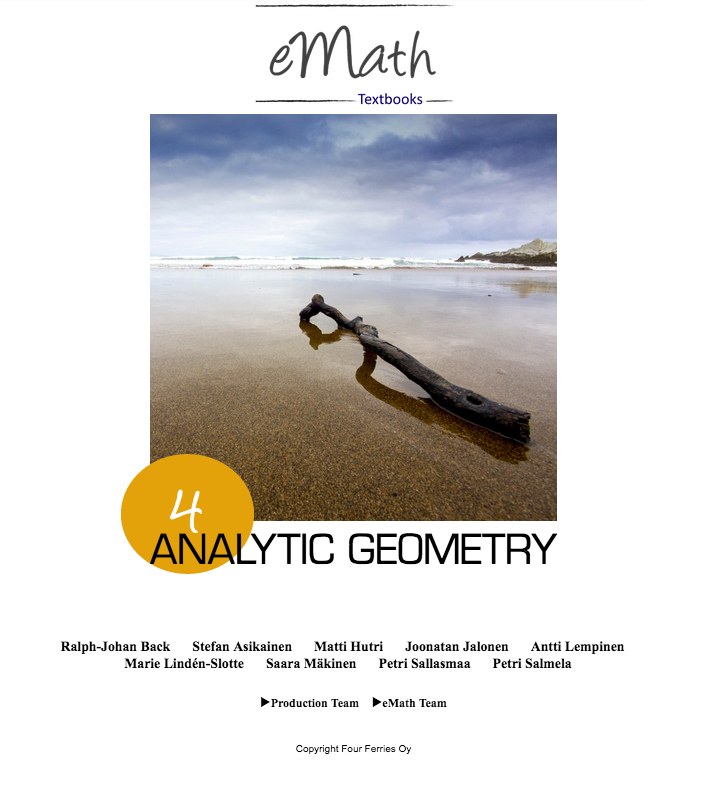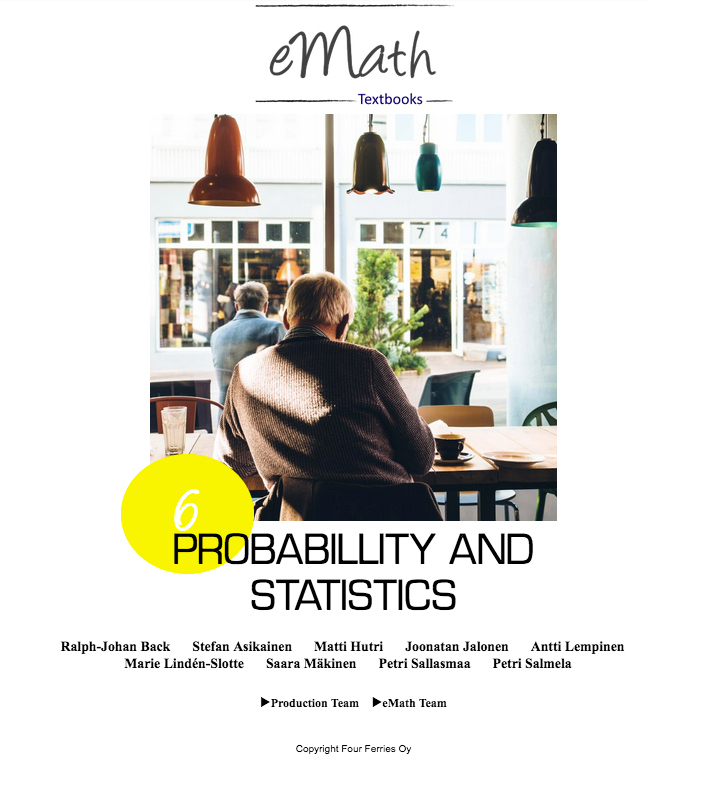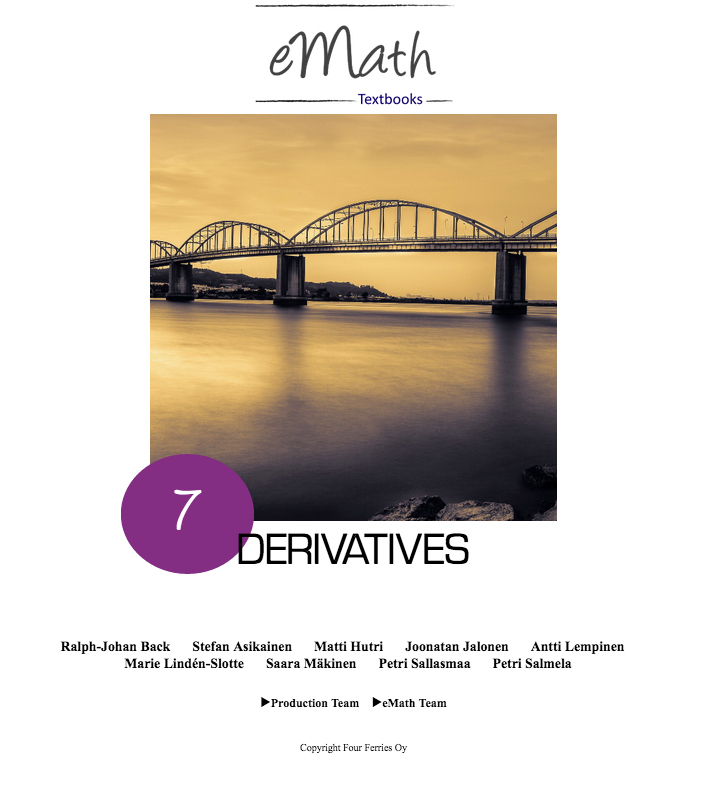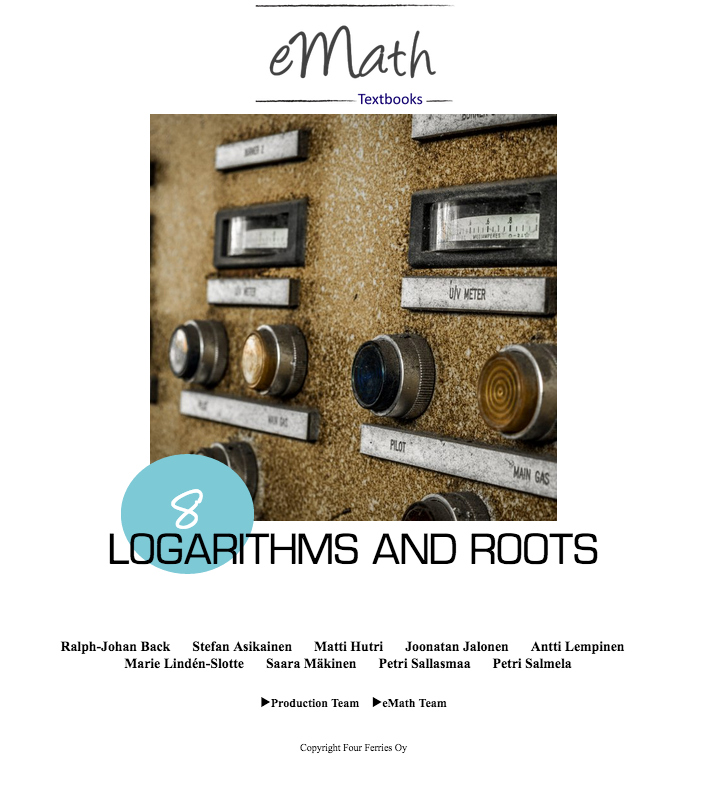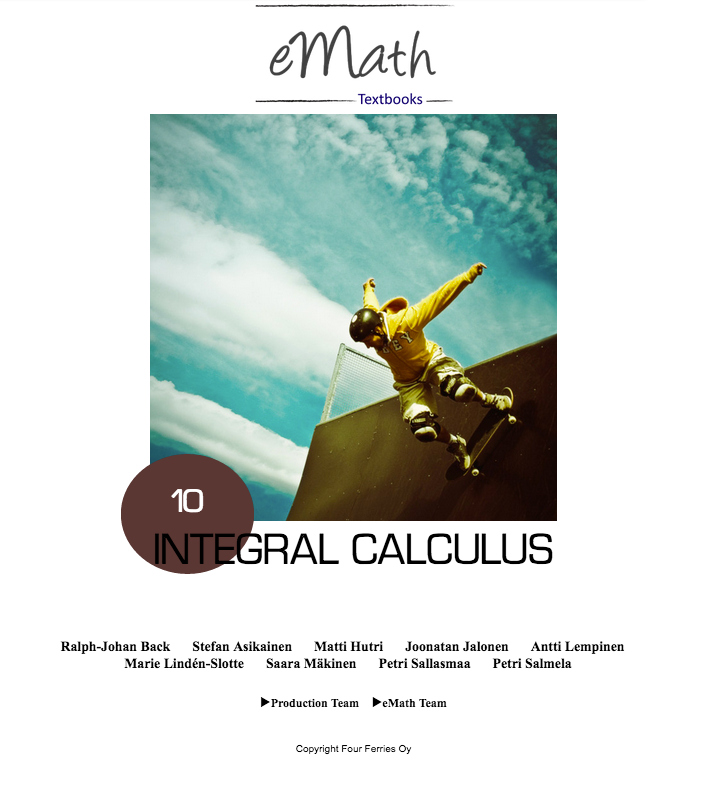Structured Derivations: Teaching Mathematical Reasoning in High school

This book is intended for mathematics teachers who want to try out the method  in practice in teaching. It shows how to structure a mathematical derivation so that it is easy to see the overall structure of the argument. The book is available at Amazon.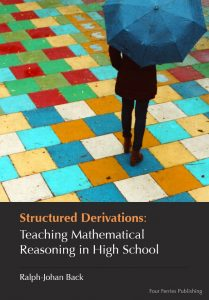### Textbooks on Structured Derivations

Four Ferries Publishing offers two textbooks on structured derivations. The first one gives an overall description of the method, and explains by a large number of examples how to use the method in practical education at high school level. The second book is a handbook on structured derivations,  covering both the method and its logical foundation. Both books are  intended for mathematics teachers on all levels of math education, to help them make their explanations easier to understand and follow.

Teaching Mathematics in the Digital Age with Structured Derivations

This book extends the first book with a more thorough treatment of the logic behind high school mathematics, as well as the logical basis of the method. It also goes further, presenting material that is directed for introductory level university mathematics. The book is available at Amazon.